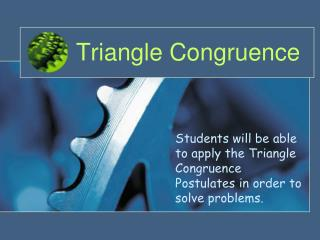DownloadDownload PresentationTriangle Congruence

# Triangle Congruence

Télécharger la présentation## Triangle Congruence

- - - - - - - - - - - - - - - - - - - - - - - - - - - E N D - - - - - - - - - - - - - - - - - - - - - - - - - - -
##### Presentation Transcript

1. Triangle Congruence Students will be able to apply the Triangle Congruence Postulates in order to solve problems.

2. Triangle Rigidity • The property of triangle rigidity gives you a shortcut for proving two triangles are congruent. • It states that if the side lengths of a triangle are given, the triangle can have only one shape. Because of this property, do we need to know all six facts (that all pairs of sides and all pairs of angles are congruent) to show that two triangles are congruent? Unit F

3. SSS SAS ASA AAS HL Do we need all six facts? • These are the five postulates or theorems that prove that two triangles are congruent using less that all six facts. NO! Unit F

4. Side-Side-Side Congruence (SSS) B D • If three sides of one triangle are congruent to three sides of another triangle, E C A F then the triangles are congruent. If , , and then Unit F

5. Included Angles • An included angle is an angle formed by two adjacent sides of a polygon. • ∠A is the included angle between and . • ∠B is the included angle between • and . • ∠Cis the included angle • between and . B C A Unit F

6. Side-Angle-Side Congruence (SAS) D • If two sides and the included angle of one triangle are congruent to two sides and the included angle of another triangle, then the triangles are congruent. B E F C A If , , and then ΔABC ≅ΔDEF Unit F

7. Included Sides • An included side is the common side of two consecutive angles in a polygon. • is the included side between ∠A and ∠B. • is the included side between • ∠B and ∠C. • is the included side • between ∠A and ∠C. B C A Unit F

8. Angle-Side-Angle Congruence (ASA) B • If two angles and the included side of one triangle are congruent to two angles and the included side of another triangle, then the triangles are congruent. D C A E F If , , and then ΔABC ≅ΔDEF Unit F

9. Angle-Angle-Side Congruence (AAS) B D • If two angles and a non-included side of one triangle are congruent to two angles and a non-included side of another triangle, then the triangles are congruent. C A E F If , , and then ΔABC ≅ΔDEF Unit F

10. Hypotenuse-Leg Congruence (HL) D B • If the hypotenuse and a leg of one right triangle are congruent to the hypotenuse and leg of another right triangle, then the triangles are congruent. E C A F If and then ΔABC ≅ΔDEF Unit F

11. SSS SAS ASA AAS HL Are there other Postulates? These are the only five Postulates or Theorems that prove that two triangles are congruent using less that all six facts. Warning! There are no Angle-Angle-Angle or Side-Side-Angle Postulates of Congruence! Unit F

12. Are there other Postulates? • This is an example of why there is noAngle-Angle-Angle Postulateof Congruence. • These two triangles have all three pairs of angles congruent, but the two triangles are not congruent. Unit F

13. Are there other Postulates? • This is an example of why there is noSide-Side-Angle Postulate of Congruence. • These two triangles have two pairs of sides and a pair of nonincluded angles congruent, but the two triangles are not congruent. Unit F

14. Name the Postulate or Theorem 1. 2. 3. 4. 5. Unit F

15. Lesson Quiz Which postulate, if any, can be used to prove the triangles congruent? 1. 2. 3. 4. Unit F